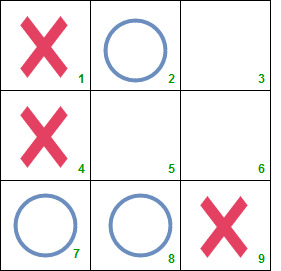# Puzzle 38 | (Tic Tac Toe Puzzle)

Statement:
The game of Tic-Tac-Toe is being played between two players and it is in below state after six moves.1. Who will win the game, O or X?
2. Which was the sixth mark and at which position?

Assume that both the players are intelligent enough.

Solution:
O will win the game. The sixth mark was X in square 9.

Explanation:
The 7th mark must be placed in square 5 which is the win situation for both X and O. Hence, the 6th mark must be placed in a line already containing two of the opponents marks. There are two such possibilities – the 6th mark would have been either O in square 7 or X in square 9.

As we know both the players are intelligent enough, the 6th mark could not be O in square 7. Instead, he would have placed O in square 5 and would have won.

Hence, the sixth mark must be X placed in square 9. And the seventh mark will be O. Thus O will win the game.